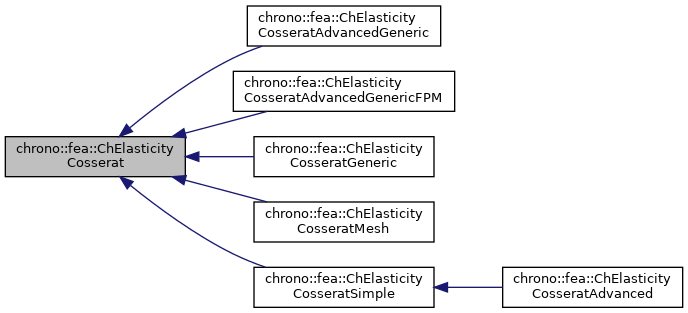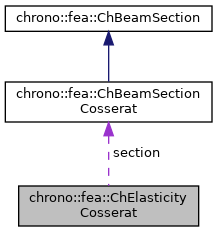chrono::fea::ChElasticityCosserat Class Referenceabstract

## Description

Base interface for elasticity of beam sections of Cosserat type, where xyz force "n" and xyz torque "m" are a 6-dimensional function of generalized strains, "e" traction/shear and "k" curvatures, as: {n,m}=f({e,k}) There are various children classes that implement this function in different ways.

Note that the Timoshenko beam theory can be a sub-case of this.

#include <ChBeamSectionCosserat.h>

Inheritance diagram for chrono::fea::ChElasticityCosserat:[legend]
Collaboration diagram for chrono::fea::ChElasticityCosserat:[legend]

## Public Member Functions

virtual void ComputeStress (ChVector<> &stress_n, ChVector<> &stress_m, const ChVector<> &strain_e, const ChVector<> &strain_k)=0
Compute the generalized cut force and cut torque, given actual deformation and curvature. More...

virtual void ComputeStiffnessMatrix (ChMatrixNM< double, 6, 6 > &K, const ChVector<> &strain_e, const ChVector<> &strain_k)
Compute the 6x6 tangent material stiffness matrix [Km] = dσ/dε, given actual deformation and curvature (if needed). More...

## Public Attributes

ChBeamSectionCosseratsection

## ◆ ComputeStiffnessMatrix()

 void chrono::fea::ChElasticityCosserat::ComputeStiffnessMatrix ( ChMatrixNM< double, 6, 6 > & K, const ChVector<> & strain_e, const ChVector<> & strain_k )
virtual

Compute the 6x6 tangent material stiffness matrix [Km] = dσ/dε, given actual deformation and curvature (if needed).

This must be overridden by subclasses if an analytical solution is known (preferred for high performance), otherwise the base behaviour here is to compute [Km] by numerical differentiation calling ComputeStress() multiple times.

Parameters
 K 6x6 material stiffness matrix values here strain_e local strain (deformation part): x= elongation, y and z are shear strain_k local strain (curvature part), x= torsion, y and z are line curvatures

## ◆ ComputeStress()

 virtual void chrono::fea::ChElasticityCosserat::ComputeStress ( ChVector<> & stress_n, ChVector<> & stress_m, const ChVector<> & strain_e, const ChVector<> & strain_k )
pure virtual

Compute the generalized cut force and cut torque, given actual deformation and curvature.

Parameters
 stress_n local stress (generalized force), x component = traction along beam stress_m local stress (generalized torque), x component = torsion torque along beam strain_e local strain (deformation part): x= elongation, y and z are shear strain_k local strain (curvature part), x= torsion, y and z are line curvatures

The documentation for this class was generated from the following files:
• /builds/uwsbel/chrono/src/chrono/fea/ChBeamSectionCosserat.h
• /builds/uwsbel/chrono/src/chrono/fea/ChBeamSectionCosserat.cpp